# Elliptic function

in the proper sense

A double-periodic function that is meromorphic in the finite complex-plane. An elliptic function has the following basic properties.

There are no entire elliptic functions except the constants (Liouville's theorem).

Let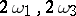be the primitive periods of an elliptic function,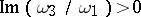(cf. Double-periodic function). The sum of the residues of all poles ofin its period parallelogram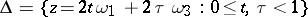is zero.

Letbe the number of poles (counted with multiplicities) of an elliptic functionin a period parallelogram. Thentakes inevery finite value exactlytimes, counted with multiplicities. The numberis called the order of. There are no elliptic functions of order less than 2.

If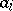and,, are all the zeros and poles of an elliptic functionin a period parallelogram, counted with multiplicities, then the sum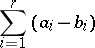is congruent to zero modulo the periods, that is,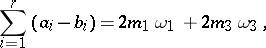where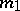and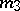are integers (a special case of Abel's theorem, see Abelian function).

All the elliptic functions with fixed primitive periodsform an algebraic field of elliptic functions with two generators. For these generators one can take, for example, the Weierstrass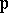-function and its derivative (see Weierstrass elliptic functions).

The derivative of an elliptic function is itself an elliptic function, having the same periods. Every elliptic function satisfies a first-order ordinary differential equation. Every elliptic functionsatisfies an algebraic addition theorem, that is, the values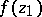,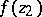and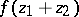are connected by an irreducible algebraic equation with constant coefficients. Conversely, Weierstrass' theorem asserts that every analytic functionadmitting an algebraic addition theorem is either a rational function ofor of, or is an elliptic function.

Sometimes a more general terminology is used, connected with the theory of theta-functions (cf. Theta-function). An elliptic function of the third kind is defined to be any meromorphic functionsatisfying a functional equation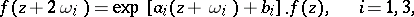whereand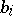are constants. If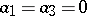, it is called an elliptic function of the second kind. If, thenis said to be of the first kind, or an elliptic function in the proper sense. In this terminology the Jacobi theta-functions (see Jacobi elliptic functions) and the Weierstrass-function (see Weierstrass elliptic functions) are of the third kind.

Elliptic integrals (cf. Elliptic integral) were first investigated in works of scholars at the end of the 17th century to the beginning of the 19th century: Jacob Bernoulli, and Johann Bernoulli, G.C. Fagnano dei Toschi, L. Euler, and A. Legendre. These integrals appeared in the problems of computing the arc length of an ellipse and other conics. They have the form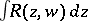, whereis a rational function in variablesandconnected by an algebraic equationin which the right-hand side is a polynomial of degree 4 or 3 without multiple roots. The integrand is single-valued on a two-sheeted compact Riemann surfaceof genus 1 with four branch points. The differentials of the first, second and third kind on(see Differential on a Riemann surface) generate elliptic integrals of the first, second and third kind, respectively. An integral of the first kind is a principal uniformizing function forand for the algebraic function field generated by. If one takes it as an independent variable, then this field becomes a field of elliptic functions.

The idea of a direct inversion of an elliptic integral in Legendre normal form originated and was developed in work of N.H. Abel and C.G.J. Jacobi at the beginning of the 19th century. The construction of elliptic functions on the basis of theta-functions, developed by Jacobi, is of fundamental importance in applications of elliptic functions. The theoretically simpler construction of the field of elliptic functions in which one takes as generators the functionand its derivative was made by K. Weierstrass in the 1870s.

One of the basic problems in the development of the theory of elliptic functions was the problem of transformation of elliptic functions and related quantities on transition from primitive periodsto other primitive periodsconnected by relations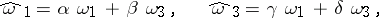whereare integers such that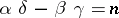, whereis natural number, the so-called order of the transformation. The area of the period parallelogram of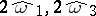istimes larger than that of the period parallelogram of. Forone obtains the transformations of the modular group, which gave rise to the theory of modular functions (cf. Modular function), connected with that of elliptic functions.

Elliptic functions can be treated as meromorphic functions that are invariant under transformations of the translation group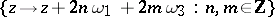of the complex plane. A generalization of this approach led to the consideration of automorphic functions (cf. Automorphic function), which are invariant under fractional-linear transformations (cf. Fractional-linear mapping), which form a group of a more general kind. Elliptic and modular functions are special cases of automorphic functions.

The inversion of elliptic integrals led straight to the Jacobi inversion problem for more general Abelian integrals (cf. Abelian integral), where the variablesand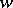are connected by an arbitrary algebraic equation. Along these lines one obtains the Abelian functions (cf. Abelian function), a generalization of elliptic functions to the case of several complex variables.

Elliptic functions and integrals have numerous applications (like special functions) in many branches of analysis, as a means of uniformization in algebraic geometry, and also in mechanics, electrodynamics, and other parts of theoretical physics.